Question

# Which of the following statements is true of weak acids dissolved in water:        They...

Which of the following statements is true of weak acids dissolved in water:

They are completely ionized in water

They are totally unionized in water

They are partially ionized in water

The dissociation constant is a function of pH

QUESTION 13

Which of the following is a hydroxide ion?

H3O+

OH-

HOOH

CH3OH2+

QUESTION 14

The following molecule is an example of which class of compounds? CH3CH2CH2COOH

Hydrocarbon

Acid

Alcohol

Aldehyde

QUESTION 15

Which of the following would not form a suitable buffer?

Acetic acid/ acetate (pKa 4.76)

Carbonic acid/ bicarbonate (pKa 3.6)

Bicarbonate/ carbonate (pKa 10.3)

Hydrochloric acid/ chloride (pKa -6.3)

1 points

QUESTION 16

How many grams of NaCl in 10 liter to make a 0.834 M solution (NaCl MW = 58.44 g / mol)?

487

QUESTION 17

The pH of a solution that is 0.25 molar in acetic acid and 0.1 molar in sodium acetate is 4.36. What is the pKa of acetic acid?

4.36

4.76

7.76

3.76

QUESTION 18

Malate dehydrogenase converts Malate to oxaloacetate in the citric acid cycle. If the ratio of products/reactants was 1/1,000,000 in this reaction in a cell, and the standard free energy change (ΔG°’) for the reaction is 29.7 kJ mole-1, what is the ΔG?

-5.6 kJ mole-1

5.6 kJ mole-1

-4.8 kJ mole-1

4.8 kJ mole-1

None of the above

QUESTION 19

What is the pH of a solution where the concentration of hydrogen ions is 2 x 10-5 molar?

5

2.5

4.7

9

QUESTION 20

Bromine is one of the few elements that exists as a liquid at room temperature. At what temperature (in K) would this reaction Br2 (l) à Br2 (g) be at equilibrium. Given that at room temperature (298 K), DH = 30.91 kJ/mol, DG = 3.13 kJ/mol and DS = 0.0932 mol.K

298 K

364 K

253 K

331 K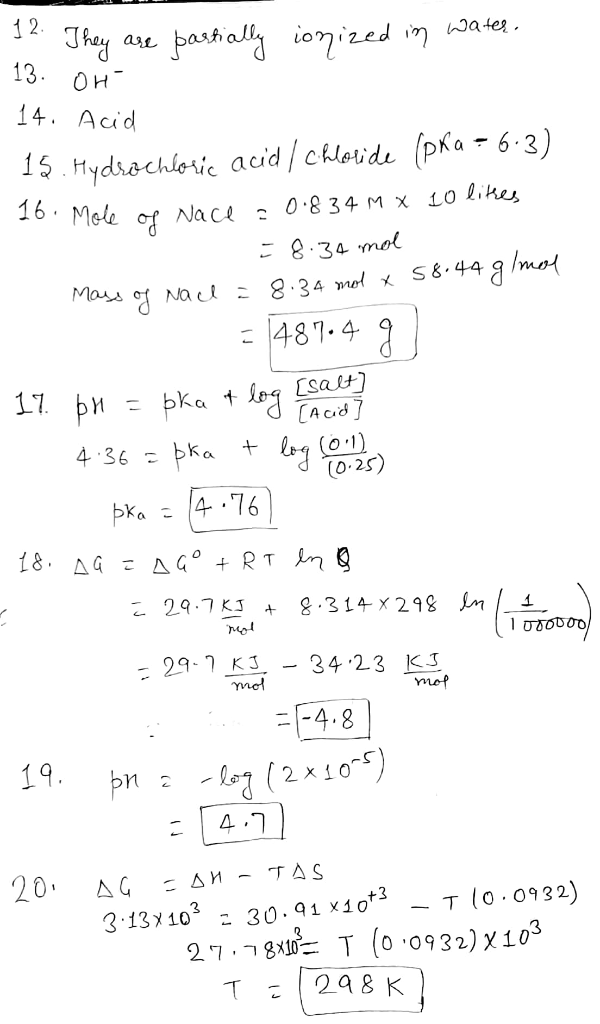#### Earn Coins

Coins can be redeemed for fabulous gifts.

Similar Homework Help Questions
• ### What concentrations of acetic acid (pKa=4.76)(pKa=4.76) and acetate would be required to prepare a 0.10 M0.10...

What concentrations of acetic acid (pKa=4.76)(pKa=4.76) and acetate would be required to prepare a 0.10 M0.10 M buffer solution at pH 4.6pH 4.6? Note that the concentration, pH value, or both may differ from that in the first question. Strategy Rearrange the Henderson–Hasselbalch equation to solve for the ratio of base (acetate) to acid (acetic acid), [A−]/[HA][A−]/[HA] . Use the mole fraction of acetate to calculate the concentration of acetate. Calculate the concentration of acetic acid. Step 1: The ratio...

• ### What concentrations of acetic acid (pKa = 4.76) and acetate would be required to prepare a...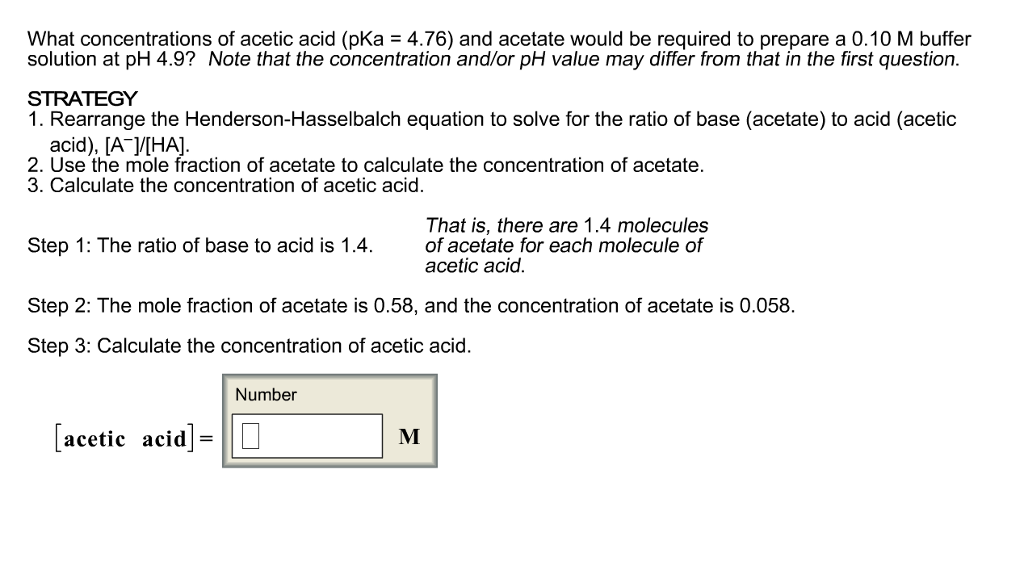What concentrations of acetic acid (pKa = 4.76) and acetate would be required to prepare a 0.10 M buffer solution at pH 4.9? Note that the concentration and/or pH value may differ from that in the first question. 1. Rearrange the Henderson-Hasselbalch equation to solve for the ratio of base (acetate) to acid (acetic acid), [A^-]/[HA]. 2. Use the mole fraction of acetate to calculate the concentration of acetate. 3. Calculate the concentration of acetic acid. That is, there are...

• ### What concentrations of acetic acid (pKa = 4.76) and acetate would be required to prepare a...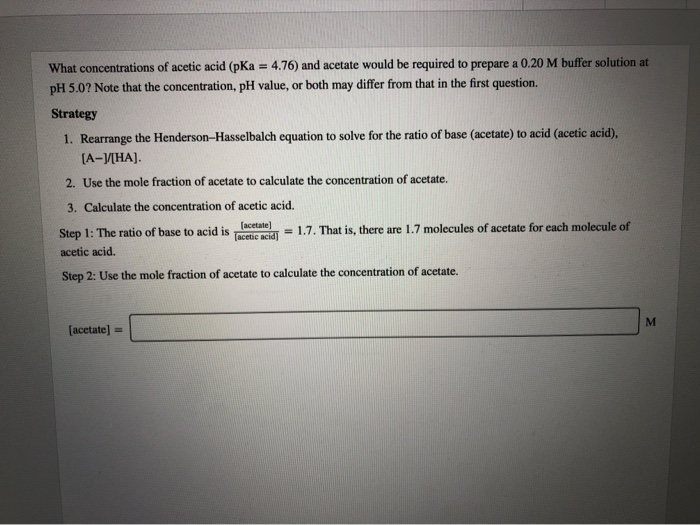What concentrations of acetic acid (pKa = 4.76) and acetate would be required to prepare a 0.20 M buffer solution at pH 5.0? Note that the concentration, pH value, or both may differ from that in the first question. Strategy 1. Rearrange the Henderson-Hasselbalch equation to solve for the ratio of base (acetate) to acid (acetic acid). [A-V[HA). 2. Use the mole fraction of acetate to calculate the concentration of acetate. 3. Calculate the concentration of acetic acid. Step 1:...

• ### 500 mg of sodium acetate is dissolved in 500.0 mL of de-ionized water. Calculate the pH...

500 mg of sodium acetate is dissolved in 500.0 mL of de-ionized water. Calculate the pH of this solution. MM(sodium acetate) = 82.0324 g/mol, Ka(acetic acid) = 1.75 x 10-5

• ### what concentration of acetic acid (pka=4.76) and acetate would be required to prepare a .15 M...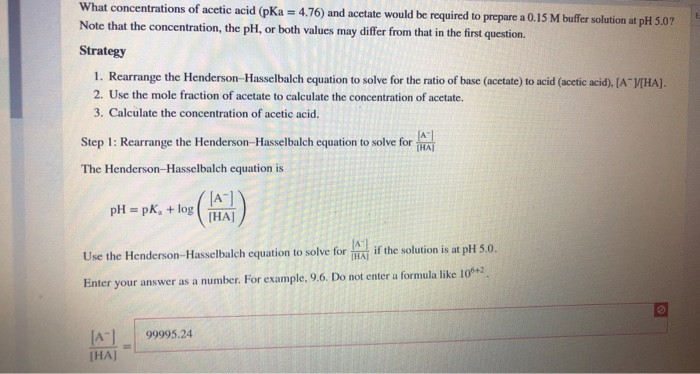what concentration of acetic acid (pka=4.76) and acetate would be required to prepare a .15 M buffer solution at pH 5.0? What concentrations of acetic acid (pKa = 4.76) and acetate would be required to prepare a 0.15 M buffer solution at pH 5.07 Note that the concentration, the pH, or both values may differ from that in the first question. Strategy 1. Rearrange the Henderson-Hasselbalch equation to solve for the ratio of base (acetate) to acid (acetic acid). (AVHA)....

• ### when a 7.00 g sample of KSCN is dissolved in water in a calorimeter that has...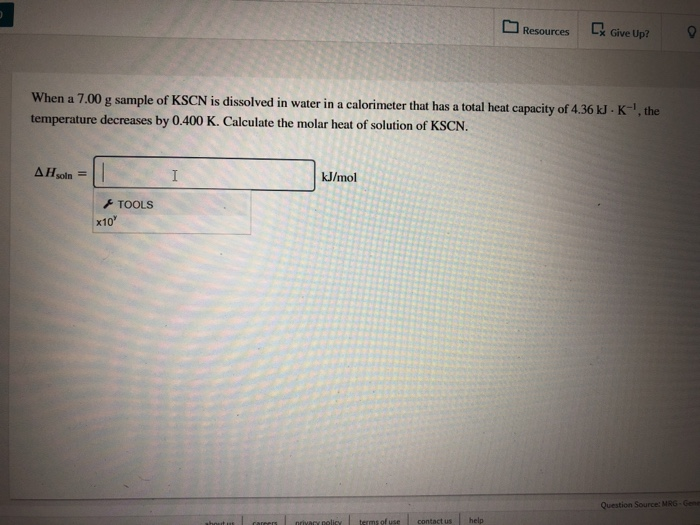when a 7.00 g sample of KSCN is dissolved in water in a calorimeter that has a total he capacity of 4.36 kJ. K^-1, the temperature decreases by .400 K. calculate the molar heat of solution of KSCN. Resources Give Up?9 When a 7.00 g sample of KSCN is dissolved in water in a calorimeter that has a total heat capacity of 4.36 kJ .K-, the decreases by 0.400 K. Calculate the molar heat of solution of KSCN kJ/mol TOOLS...

• ### What concentrations of acetic acid (pKa = 4.76) and acetate would be required to prepare a...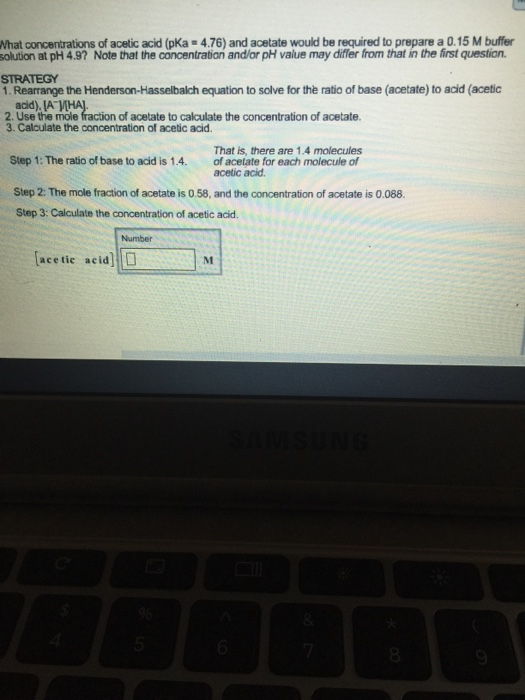What concentrations of acetic acid (pKa = 4.76) and acetate would be required to prepare a 0.15 M buffer solution at pH 4.9? Note that the concentration and/or pH value may differ from that m the first question. STRATEGY 1. Rearrange the Hendereon-Hasselbalch equation to solve for thfe rabo of base (acetate) to aad (acetic add).[A^-]/[HA]. 2. Use the mole fraction of acetate to calculate the concentration of acetate. 3. Calculate the concentration ot acetic acid.

• ### 1. 200 mL of an aqueous solution contains 0.030 M concentrations of both Pb2+ and Ag+....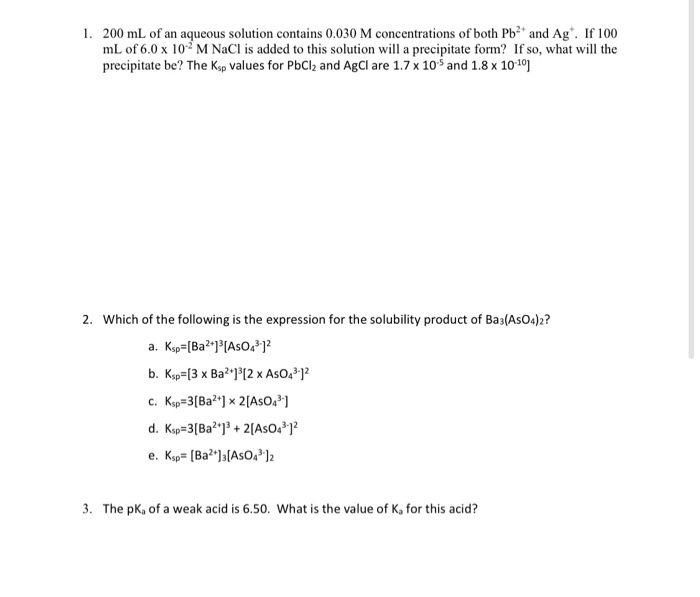1. 200 mL of an aqueous solution contains 0.030 M concentrations of both Pb2+ and Ag+. If 100 mL of 6.0 x 10-2 M NaCl is added to this solution will a precipitate form? If so, what will the precipitate be? The Ksp values for PbCl2 and AgCl are 1.7 x 10-5 and 1.8 x 10-10] 2. Which of the following is the expression for the solubility product of Ba3(AsO4)2? 3. The pKa of a weak acid is 6.50. What...

• ### 4. A solution is made by dissolving 15.00 grams of acetic acid and 3.25 grams of...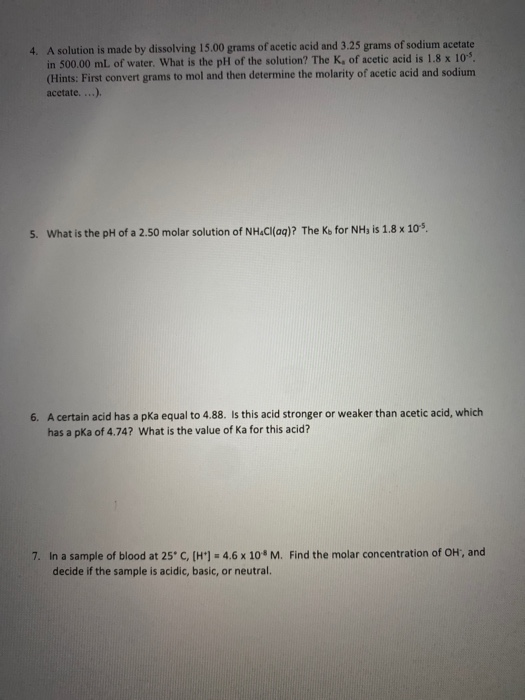4. A solution is made by dissolving 15.00 grams of acetic acid and 3.25 grams of sodium acetate in 500.00 mL of water. What is the pH of the solution? The K, of acetic acid is 1.8 x 10. (Hints: First convert grams to mol and then determine the molarity of acetic acid and sodium acetate, ...) 5. What is the pH of a 2.50 molar solution of NHCl(aq)? The Ko for NH, is 1.8 x 105. 6. A certain...

• ### 1. 1x10^-9 moles of strong acid HCl are added to of water (total volume is 1L)....

1. 1x10^-9 moles of strong acid HCl are added to of water (total volume is 1L). the pH of the resulting solution is... a)9 b)8 c)7 d)5 e)2 I got 9 but got the answer incorrect, so i would like to know how to solve this properly and what is the final answer. 2. the pKa of acetic acid is 4.76. if the pH=4.76 and the concentration of sodium acetate is 0.1M, what is the concentration of acetic acid? a)0.05...